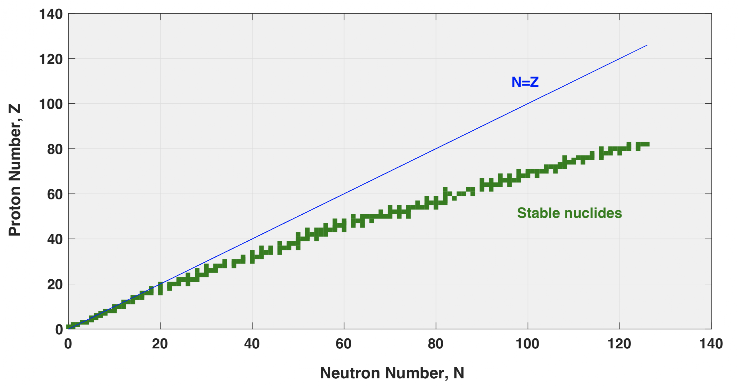Nuclear Instability
Test your knowledge of nuclear instability
What school or college do you attend? *
Element type is determined by which of the following? *
1 point
Which of the following describes beta (minus) decay? *
1 point
The picture shows a plot of graph of N against Z, with the green region indicating the stable nuclides. It doesn't follow the N=Z line, why do you think that is? *
1 pointIn alpha decay, how many protons and how many neutrons are removed from the decaying nucleus? *
1 point
Alpha decays can be used as a fingerprint to identify decays, why is this?
1 point
A sequence of unstable atomic nuclei and their modes of decays, which lead to a stable nucleus, is known as what? *
1 point
Which of these describes how the number of radioactive nuclei in a sample decay as a function of time? *
1 point
When measuring the activity of a radioactive sample it is important to observe many decays in the experiment, why is this? *
1 point
An experiment is being undertaken to study “proton-rich” nuclei that have an imbalance in the ratio of protons and neutrons. Which of the following decay types do you think is most likely to be observed to rectify the imbalance in the nucleons ratio? *
1 point
The half-life of an unknown nuclear sample is 5 years. If there are 200grams initially in the sample, how much will be left after 15 years?
1 point